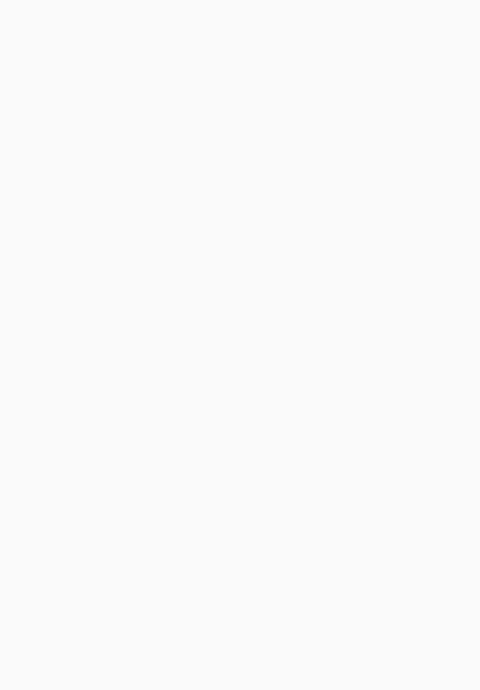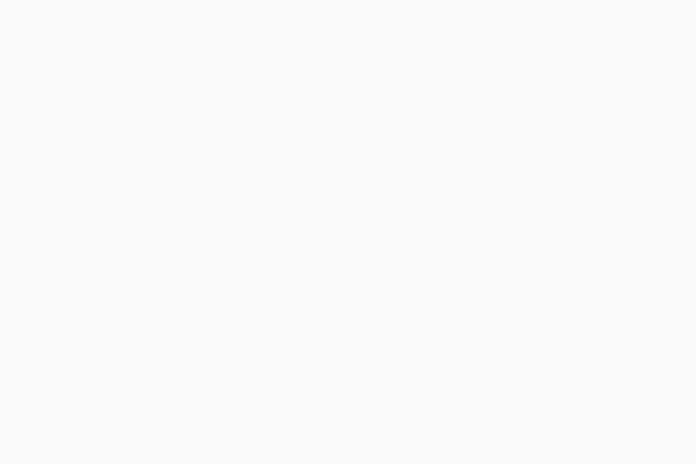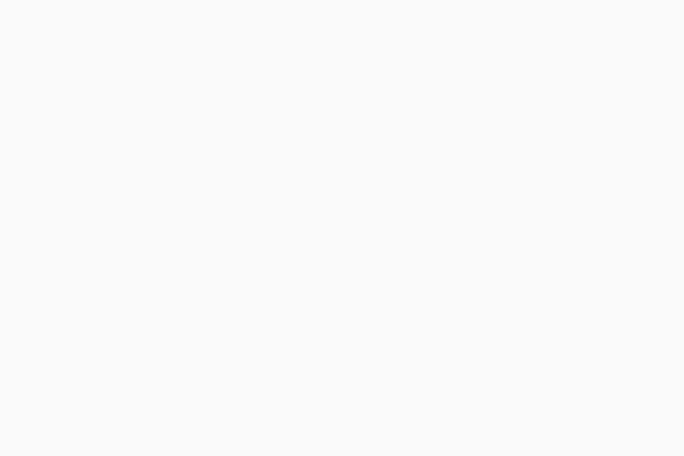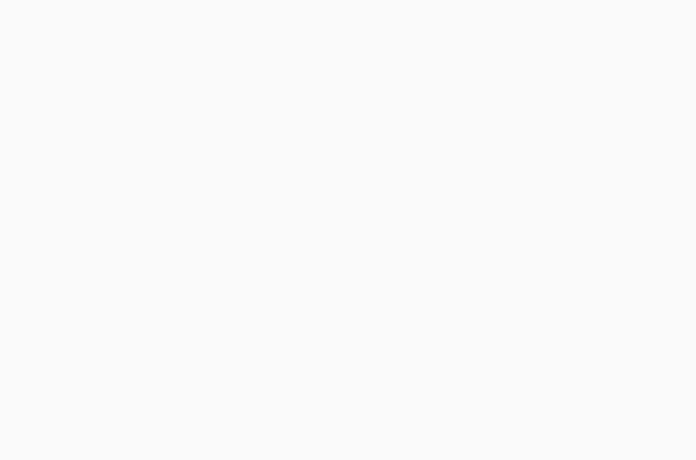# How Many Shingles In A Bundle | All You Need To Know On Roof Construction

Roof construction takes a lot of preparation and calculation in order to be successful. One of the most important preparations in roof construction or remodeling is the estimation of the amount of shingles that you will need. On that note, we offer this quick article on shingle estimation, as well as to answer the question: how many shingles are there in a bundle?

More...

## How Do You Measure the Surface Area of Your Roof?

Before we start determining the amount of shingles you need, let’s talk about the ways on how you can measure your roof to get the total surface area.The easiest and most accurate way you can do this is to measure the length and width of your roof or the particular area wherein you will need remodeling. When you have acquired these values, simply multiply the length and the width to get the square footage of each roof plane.

SAFETY TIP

Measure the width of the building instead to get the width of your roof, if applicable, from the ground. Then, measure the height (from the eaves to the ridge) from a ladder while using a metal measuring tape.

Another method you can use is the sheath-count method. This is applicable when the sheathing is still exposed, and can be done from the ground.

This is done by counting the number of shingles from left to right in order to get the width of the section in feet. One 3-tab shingle is approximately 12 x 6” in measurement so you can estimate the value from this.

After getting the width of your section, you can then count the number of rows of shingles between the eaves to the ridge. Divide this result by 2 to get the height of your roof.

Similarly, you can multiply the height and width to get the total surface area of your roof.

## How Many Shingles in a Bundle?Now that you’ve determined the surface area of your roof to be remodeled or constructed, it’s time to buy your shingles. How do we do that?

What you’ll need to know is that a square comprises of three bundles. In one bundle, there are 29 standard sized shingles. Note that the standard size of a shingle is measured at 12 x 36”.

## How Many Bundles Do You Need to Buy?

From our roof measurements, we know the total square footage of our roof. To determine the number of squares, divide that value by 100. By this point, we know that a square is equal to three bundles.Therefore, for a 100 sq feet roof, we need approximately one square or three bundles of shingles. With a bundle consisting of 29 shingles, we can tell that a 100 sq. ft. roof will need about 87 shingles.

TIP

Account for the amount of waste by adding 10% to the amount of shingles that you will need. This will ensure that you will not fall short on shingles, as well as make sure that you have enough when cutting them to fit into the ridge.

## Conclusion

Estimating the amount of shingles that you will need is pretty simple, and can be done from the ground. Here’s a rundown of the steps you need to do:

• Measure the surface area of your roof
• Using the surface area in square feet, divide this number by 100 to come up with the number of squares
• Divide the amount of squares by 3 to get the number of bundles
• Multiply the number of bundles by 29 to get the number of shingles
• Add 10% to account for the waste factorSee? Easy as pie. Moreover, roof construction or remodeling will be much easier with the proper tools, such as the best framing hammer for the job.

•